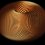# Square root

hello , I really urgently need your help in this problem The product of 2 natural nos. is 15120 and their HCF is 6.Find how many such pairs exist.

Pls help me to find the solution to this problemNote by Erica Phillips
3 years, 7 months ago

This discussion board is a place to discuss our Daily Challenges and the math and science related to those challenges. Explanations are more than just a solution — they should explain the steps and thinking strategies that you used to obtain the solution. Comments should further the discussion of math and science.

When posting on Brilliant:

• Use the emojis to react to an explanation, whether you're congratulating a job well done , or just really confused .
• Ask specific questions about the challenge or the steps in somebody's explanation. Well-posed questions can add a lot to the discussion, but posting "I don't understand!" doesn't help anyone.
• Try to contribute something new to the discussion, whether it is an extension, generalization or other idea related to the challenge.

MarkdownAppears as
*italics* or _italics_ italics
**bold** or __bold__ bold
- bulleted- list
• bulleted
• list
1. numbered2. list
1. numbered
2. list
Note: you must add a full line of space before and after lists for them to show up correctly
paragraph 1paragraph 2

paragraph 1

paragraph 2

[example link](https://brilliant.org)example link
> This is a quote
This is a quote
    # I indented these lines
# 4 spaces, and now they show
# up as a code block.

print "hello world"
# I indented these lines
# 4 spaces, and now they show
# up as a code block.

print "hello world"
MathAppears as
Remember to wrap math in $$ ... $$ or $ ... $ to ensure proper formatting.
2 \times 3 $2 \times 3$
2^{34} $2^{34}$
a_{i-1} $a_{i-1}$
\frac{2}{3} $\frac{2}{3}$
\sqrt{2} $\sqrt{2}$
\sum_{i=1}^3 $\sum_{i=1}^3$
\sin \theta $\sin \theta$
\boxed{123} $\boxed{123}$

Sort by:

Hint: Let $A$ and $B$ be the 2 integers. Show that $\dfrac A6$ and $\dfrac B6$ are coprime.

Hint 2: Factorize $\dfrac{15120}{6\times6}$ into product of 2 coprime posiitve integers.

- 3 years, 7 months ago

I have factorized 420 by 7 and 5 and then??

- 3 years, 7 months ago

We know that $A/6$ and $B/6$ are coprime positive integers, and that $A/6 \times B/6 = 420$.

So we want to find 2 coprime positive integers that give a product of 420.

For example $1\times 420$, $4\times 105$, $3\times35$, $15 \times 7$.

Is there any other way?

- 3 years, 7 months ago

I just wanna ask why have you divided A and B by 6 to prove that they are co prime??

- 3 years, 7 months ago

Both $A$ and $B$ are divisible by 6, right?

Are there any other larger integer that must divide both $A$ and $B$?

- 3 years, 7 months ago

but why is it 6??

- 3 years, 7 months ago

... The product of 2 natural nos. is 15120 and their HCF is 6

In other words, the largest integer that divides both $A$ and $B$ is....?

- 3 years, 7 months ago

oh ok thanx !!

- 3 years, 7 months ago

so u wanna say that 1420,4105,335,157 are the probable pairs.right??

- 3 years, 7 months ago

No. $\quad \quad$

- 3 years, 7 months ago